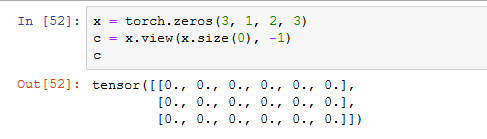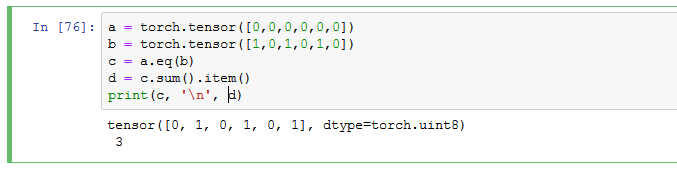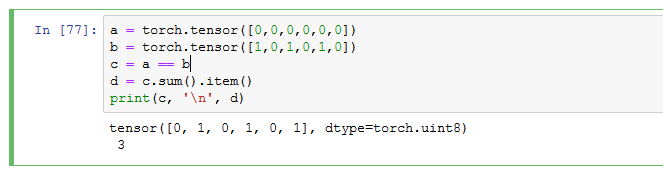# pytorch训练kaggle猫狗大战分类器

Posted by kevin on March 18, 2020

## 划分数据集

from torchvision.models.resnet import resnet18
import os
import random
from PIL import Image
import torch.utils.data as data
import numpy as np
import torchvision.transforms as transforms
import torch
import torch
import torch.nn as nn
import torch.optim as optim
from torch.optim.lr_scheduler import *
import torchvision.transforms as transforms
import numpy as np
import os


class DogCat(data.Dataset):
def __init__(self, root, transform=None, train=True, val=False):
self.val = val
self.train = train
self.transform = transform
# imgs为一个储存了所有数据集绝对路径的列表
imgs = [os.path.join(root, img) for img in os.listdir(root)]

if self.val:
imgs = sorted(imgs, key=lambda x: int(x.split('.')[-2].split('/')[-1]))
else:
# 根据图片的num排序，如 cat.11.jpg -> 11
imgs = sorted(imgs, key=lambda x: int(x.split('.')[-2]))
imgs_num = len(imgs)
random.shuffle(imgs) # 打乱顺序
if self.train:
self.imgs = imgs[:int(0.7 * imgs_num)]
else:
self.imgs = imgs[int(0.7 * imgs_num):]

# 作为迭代器必须有的方法
def __getitem__(self, index):
img_path = self.imgs[index]
label = 1 if 'dog' in img_path.split('/')[-1] else 0  # 狗的label设为1，猫的设为0
data = Image.open(img_path)
data = self.transform(data)
return data, label

def __len__(self):
return len(self.imgs)


torchvision.transforms.Compose 类里有很多针对图像的变化，包括图像旋转以及随即裁剪等增强方式，注意我们这里输入的图片是三维的 PIL image，所以要用 ToTensor() 方法将其转化成 pytorch 的 tensor 形式

# 对数据集训练集的处理，其实可以直接放到 DogCat 类里面去
transform_train = transforms.Compose([
transforms.Resize((256, 256)),  # 先调整图片大小至256x256
transforms.RandomCrop((224, 224)),  # 再随机裁剪到224x224
transforms.RandomHorizontalFlip(),  # 随机的图像水平翻转，通俗讲就是图像的左右对调
transforms.ToTensor(), # Convert a PIL Image or numpy.ndarray to tensor.
transforms.Normalize((0.485, 0.456, 0.406), (0.229, 0.224, 0.2225))  # 归一化，数值是用ImageNet给出的数值
])

# 对数据集验证集的处理
transform_val = transforms.Compose([
transforms.Resize((224, 224)),
transforms.ToTensor(),
transforms.Normalize((0.485, 0.456, 0.406), (0.229, 0.224, 0.225)),
])


# 生成训练集和验证集
trainset = DogCat('/data/rpcv/kevin/dataset/dogs-vs-cats-redux-kernels-edition/train', transform=transform_train)
valset = DogCat('/data/rpcv/kevin/dataset/dogs-vs-cats-redux-kernels-edition/train', transform=transform_val, train=False, val=True)
# 将训练集和验证集放到 DataLoader 中去，shuffle 进行打乱顺序（在多个 epoch 的情况下）
# num_workers 加载数据用多少的子线程（windows不能用这个参数）


## 定义网络class Net(nn.Module):
def __init__(self, model):
super(Net, self).__init__()
# 去掉model的最后1层
self.resnet_layer = nn.Sequential(*list(model.children())[:-1])
self.Linear_layer = nn.Linear(512, 2) #加上一层参数修改好的全连接层

def forward(self, x):
x = self.resnet_layer(x)
x = x.view(x.size(0), -1)
x = self.Linear_layer(x)
return x


## 定义训练测试函数

def train(epoch):
print('\nEpoch: %d' % epoch)
#     scheduler.step()
model.train()
train_acc = 0.0
for batch_idx, (img, label) in enumerate(trainloader): # 迭代器，一次迭代 batch_size 个数据进去
image = img.to(device)
label = label.to(device)
out = model(image)
loss = criterion(out, label)
loss.backward()
optimizer.step()
train_acc = get_acc(out, label)
print("Epoch:%d [%d|%d] loss:%f acc:%f" % (epoch, batch_idx, len(trainloader), loss.mean(), train_acc))


def val(epoch):
print("\nValidation Epoch: %d" % epoch)
model.eval()
total = 0
correct = 0
for batch_idx, (img, label) in enumerate(valloader):
image = img.to(device)
label = label.to(device)
out = model(image)

_, predicted = torch.max(out.data, 1)

total += image.size(0)
correct += predicted.data.eq(label.data).cpu().sum()
print("Epoch:%d [%d|%d] total:%d correct:%d" % (epoch, batch_idx, len(valloader), total, correct.numpy()))
print("Acc: %f " % ((1.0 * correct.numpy()) / total))def get_acc(output, label):
total = output.shape
_, pred_label = output.max(1)
num_correct = (pred_label == label).sum().item()
return num_correct / total## 主函数

if __name__ =='__main__':
resnet = resnet18(pretrained=True) # 直接用 resnet 在 ImageNet 上训练好的参数
device = torch.device('cuda' if torch.cuda.is_available() else 'cpu')  # 若能使用cuda，则使用cuda
model = Net(resnet) # 修改全连接层
# print(model) # 打印出模型结构
model = model.to(device) # 放到 GPU 上跑
optimizer = torch.optim.SGD(model.parameters(), lr=0.001, momentum=0.9, weight_decay=5e-4)  # 设置训练细节
criterion = nn.CrossEntropyLoss() # 分类问题用交叉熵普遍
for epoch in range(2):
train(epoch)
val(epoch)
torch.save(model, 'modelcatdog.pt')  # 保存模型


## detect.py

import torch
import cv2
import torch.nn as nn
import torch.nn.functional as F
import matplotlib.pyplot as plt
# from dogcat import Net  ##重要，若没有引入这个模型代码，加载模型时会找不到模型
from torchvision import transforms
from PIL import Image


classes = ('cat', 'dog')
if __name__ == '__main__':
device = torch.device('cuda' if torch.cuda.is_available() else 'cpu')
model = torch.load('/data/rpcv/kevin/code/jupyter/modelcatdog.pt')  # 加载模型
model = model.to(device)
model.eval()  # 把模型转为test模式
img = cv2.imread("/data/rpcv/kevin/dataset/dogs-vs-cats-redux-kernels-edition/test/1.jpg")  # 读取要预测的图片
plt.imshow(cv2.cvtColor(img, cv2.COLOR_BGR2RGB))
plt.show()
img = Image.fromarray(cv2.cvtColor(img, cv2.COLOR_BGR2RGB))
trans = transforms.Compose([
transforms.Resize((224, 224)),
transforms.ToTensor(),
transforms.Normalize((0.485, 0.456, 0.406), (0.229, 0.224, 0.225)),
])

img = trans(img)
img = img.to(device)
img = img.unsqueeze(0)  # 图片扩展多一维,因为输入到保存的模型中是4维的[batch_size,通道,长，宽]，而普通图片只有三维，[通道,长，宽]
output = model(img)
prob = F.softmax(output, dim=1)  # prob是2个分类的概率
value, predicted = torch.max(prob, 1) # torch.max 返回最大值和最大值的索引号
pred_class = classes[predicted.item()]
print('predicted class is {}, probability is {}%'.format(pred_class, round(value.item(), 6) * 100))